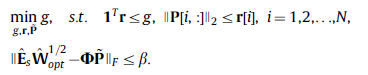# New comer in SOCP Problem

Hello! This is a newcomer in CVX. Can anybody recommend some materials about how to solve SOCP problem. Here is an example.
https://sci-hub.tw/10.1016/j.sigpro.2012.03.020

We want to solve the problem in the attached paper in Eq.15. Currently, I am getting in trouble with the F-norm constraint.

It may be easy for an expert but nor for a new one.

Appreciate it very much.

Can you enter the equation here, complete with all assumptions, explanation of notation, specification of which variables are decision (optimization) variables vs. input data?

Thanks, Mark. Here is the problem description.In the problem, Matrix P with dimension NxQ is unknown.
r is a column vector with size N. r is actually an intermediate variable.
Es and Wopt are known matrices with dimension MxK and KxK. \Phi is also known as Mxk. \Beta is a known positive priori.
Our goal is to solve matrix P.

Thanks again for your help. If you have any questions, please feel free to post it.

Thanks.

Ignoring hats, tilde, etc, and presuming `sqrtm(Wopt)` is W_{opt}^{1/2}, use
`norm(E*sqrt(mWopt) - Phi*P,'fro') <= beta`

I recommend you read or re-read the CVX Users’ Guide http://cvxr.com/cvx/doc/ In particular, have you read http://cvxr.com/cvx/doc/funcref.html#nonlinear ?

THANKS Mark. I did read those materials. I will try according to your comments.I meant `sqrtm(Wopt)`, not `sqrt(Wopt)`.I’ve now corrected it.

Is sqrtm() also a built-in function in CVX. I did not find it actually in the built-function list given by http://cvxr.com/cvx/doc/funcref.html#built-in-functions?

`sqrtm` is NOT a CVX function. It is a MATLAB function which can be applied to MATLAB variables, not to CXV variables or expressions. You wrote that `Wopt` is a known matrix, i.e., input data., so therefore, MATLAB functions can be applied to it. I wrote
presuming `sqtm(Wopt)` is W_{opt}^{1/2}
because I was leaving it to you to create whatever input matrix is needed there.That has nothing to do with CVX.

OK, get it. I will have a try first. Hope it works. Thanks.